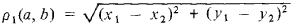# metric

(redirected from -metric)
Also found in: Dictionary, Thesaurus, Medical, Financial.

## metric

1. of or relating to the metre or metric system
2. Maths denoting or relating to a set containing pairs of points for each of which a non-negative real number ρ(x, y) (the distance) can be defined, satisfying specific conditions
3. Maths the function ρ(x, y) satisfying the conditions of membership of such a set (a metric space)
Collins Discovery Encyclopedia, 1st edition © HarperCollins Publishers 2005
The following article is from The Great Soviet Encyclopedia (1979). It might be outdated or ideologically biased.

## Metric

a mathematical term that denotes the rule for determining a given distance between any two points (elements) of a given set A. A real number function that satisfies the following three conditions is called the distance ρ (a, b) between the points a and b of the set A: (1) ρ (a, b) ≧ 0, where ρ(a, b) = 0 if and only if a = b; (2) ρ(a, b) = ρ(b, a); and (3) ρ(a, b) + ρ(b, c) ≧ ρ(a, c). For a given set M, a metric may be introduced in other ways. For example, on a plane we may take not only the ordinary Euclidean distanceas the distance between points a and b having coordinates (x1, y1) and (x2, y2), respectively, but we may take other distances as well, for example,

ρ1(a, b) = ǀx1x2ǀ + ǀy1y2ǀ

In (functional and coordinate) vector spaces a metric is often defined as a norm or, sometimes, as a scalar product. In differential geometry, a metric is introduced by specifying an element of arc length by means of a differential quadratic form. A set that has a metric introduced in it is referred to as a metric space.

A metric is sometimes understood to denote a rule for determining not only distances but also angles; an example is a projective metric.

V. I. SOBOLEV

## metric

[′me·trik]
(mathematics)
A real valued “distance” function on a topological space X satisfying four rules: for x, y, and z in X, the distance from x to itself is zero; the distance from x to y is positive if x and y are different; the distance from x to y is the same as the distance from y to x ; and the distance from x to y is less than or equal to the distance from x to z plus the distance from z to y (triangle inequality).
McGraw-Hill Dictionary of Scientific & Technical Terms, 6E, Copyright © 2003 by The McGraw-Hill Companies, Inc.